## TN State Board 11th Computer Science Important Questions Chapter 10 Flow of Control

Question 1.
What is control flow?
The flow of control jumps from one part of the code to another segment of code. This is called as “control flow.”

Question 2.
How many kinds of statements are used in C++?
There are two kinds of statements used in C++.

1. Null statement
2. Compound statementQuestion 3.
What do you mean by sequential statement?

1. The sequential statement are the statements, that are executed one after another only once from tqp to bottom.
2. They always end with a semicolon (;).

Question 4.
Write the general Syntax of the if statement.
if (expression)
true-block;
statement-x;

Question 5.
Write the three forms of nested if.

1. If nested inside if part
2. If nested inside else part
3. If nested inside both if part and else part.Question 6.
Write the Syntax of the conditional operator.
The syntax of the conditional operator is: expression 1? expression 2 : expression 3

Question 7.
What is iteration statement?

1. An iteration (or looping) is a sequence of one or more statements that are repeatedly executed until a condition is satisfied.
2. These statements are also called as control flow statements.

Question 8.
What are the types of iteration statements?
C++ supports three types of iteration statements. They are:

1. for statement
2. while statement
3. do-while statement.Question 9.
What are the four elements of a loop?
Every loop has four elements that are used for different purposes. These elements are

1. Initialization expression
2. Test expression
3. Update expression
4. The body of the loop.

Question 10.
State reason to prefer prefix operator over postfix.
The update expression contains increment/ decrement operator (++ or – -). In this part, always prefer prefix increment/decrement operator over postfix when to be used alone. The reason behind this is that when used alone, prefix operators are executed faster than postfix.

Question 11.
What is jump statement? Write the types of Jump statements are used to interrupt the normal flow of program.
Types of Jump Statements are:

1. goto statement
2. break statement
3. continue statement.Question 12.
What is goto statement? Write the Syntax.
The goto statement is a control statement which is used to transfer the control from one place to another place without any condition in a program.
The syntax of the goto statement is;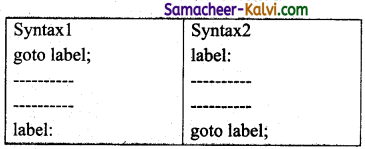Question 13.
When will the while loop be infinite? Give example.
A while loop may be infinite loop when no update statement inside the body of the loop. Eg:
int main( )
{
int i = 0;
while(i<=10)
cout<<“The value of i is”
<<i; → [This statement is infinitely displaying because no update statement inside body of the loop]
i++; → [This is not part of the loop statement because of missing curly braces]
return 0;
}

Question 14.
What is the use of update expression?
Update expression is used to change the value of the loop variable. This statement is executed at the end of the loop after the body of the loop is executed.Question 15.
Write short note on iteration statement.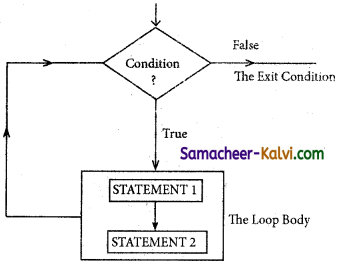The iteration statement is a set of statement that are repetitively executed if the condition is true. As soon as the condition becomes false, the repetition stops. This is also known as looping statement or iteration statement.
The set of statements that are executed again and again is called the body of the loop. The condition on which the execution or exit from the loop is called exit-condition or test- condition.

Question 16.
What do you mean by If-else-if ladder?
The if-else ladder is a multi-path decision making statement.
if(expression 1)
{
Statement-1
}
else
if (expression 2)
{
Statement-2
}
else
if (expression 3)
{
Statement-3
}
else
{
Statement-4
}

When expression becomes true, the statement associated with block is executed and the rest of the ladder is bypassed. If none of the conditions is true, then the final else statement will be executed.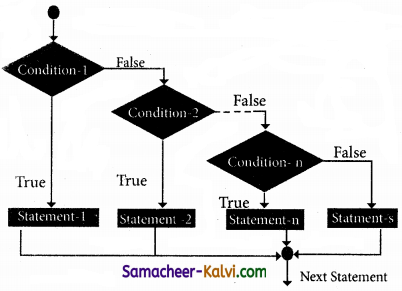Question 17.
#include
using namespace std;
int main( )
{
int marks;
cout<<“Enter the Marks ; cin>>marks;
if(marks >= 60)
cout<<“Your grade is 1st class !!” <<endl; else if(marks >= 50 && marks < 60)
cout<<“your grade is 2nd class ! ! ” <<endl; else if(marks >= 40 && marks < 50)
else
cout<<“You are fail !!”<<endl;
return 0;
}
Output:
Enter the Marks :60

Question 18.
List down the rules for the switch statement?

1. The expression provided in the switch should result in a constant value otherwise it would not be valid.
2. Duplicate case values are not allowed.
3. The default statement is optional.
4. The break statement is used inside the switch to terminate a statement sequence.
5. The break statement is optional. If omitted, execution will continue on into the next case.
6. Nesting of switch statements is also allowed.

Question 19.
Explain how conditional operator works with example?
(i) The conditional operator (or Ternary operator) is an alternative for ‘if else statement’.
(ii) The conditional operator consists of two symbols (?:). It takes three arguments.
The syntax of the conditional operator is: expression 1 ? expression 2 : expression 3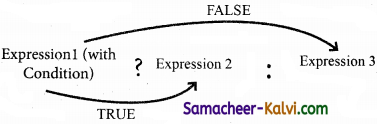Eg:
#include
using namespace std;
int main( )
{
int a, b, largest;
cout<<“\n Enter any two numbers:” ; cin>> a >> b;
largest = (a>b)? a : b;
cout<< “\n Largest number :”<< largest;
return 0;
}
Output:
Enter any two numbers: 12 98
Largest number : 98Question 20.
Write some important points about switch statement.

1. A switch statement can only work for quality of comparisons.
2. No two case labels in the same switch can have identical values.
3. If character constants are used in the switch statement, they are automatically converted to their equivalent ASCII codes.
4. The switch statement is more efficient choice than if in a situation that supports the nature of the switch operation.
The switch statement is more efficient than if-else statement.

Question 21.
Write the while loop syntax and the control flow.
(i) A while loop is a control flow statement that allows the loop statements to be executed as long as the condition is true.
(ii) The while loop is an entry-controlled loop because the test-expression is evaluated before entering into a loop.
The while loop syntax is:
while(Test expression)
{
Body of the loop;
}
Statement-x;
The control flow and flow chart of the while loop is shown below.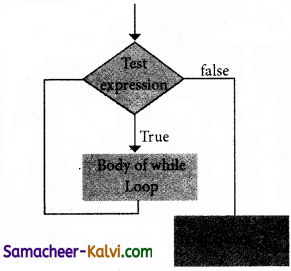while loop control flow and while loop flowchart.

Question 22.
What do you mean by empty loop?
Empty loop means a loop has no statement in its body is called an empty loop. Following for loop is an empty loop:
for (i+0; i<=5; +=i)(;) → the body of for loop contains a null statement.
In the above statement, the for loop contains a null statement, it is an empty loop.Question 23.
What do you mean by nested switch? Write the Syntax.
(i) When a switch is a part of the statement sequence of another switch, then it is called as nested switch statement.
(ii) The inner switch and the outer switch constant may or may not be the same.
The syntax of the nested switch statement is:
switch(expression)
{
case constant 1:
statement(s);
break;
switch(expression)
{
case constant 1:
statement (s);
break;
case constant 2:
statement(s);
break;
.
.
.
default :
statement(s);
}
case constant 2:
statement(s);
break; .
default :
statement (s);
}

Question 24.
How will you give multiple initialization and multiple update expressions in for loop?
(i) Multiple statements can be used in the initialization and update expressions of for loop.
(ii) These multiple initialization and multiple update expressions are separated by commas.
Eg: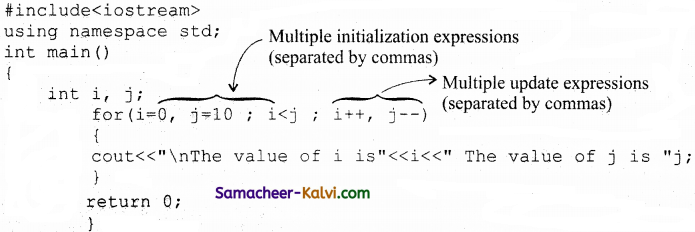Output:
The value of i is 0 The value of j is 10
The value of i is 1 The value of j is 9
The value of i is 2 The value of j is 8
The value of i is 3 The value of j is 7
The value of i is 4 The value of j is 6Question 25.
Write a short note on break with and show the working of break with different looping statements.
A break statement is a jump statement which terminates the execution of loop and the control is transferred to resume normal execution after the body of the loop. The following Figure, shows the working of break statement with looping statements: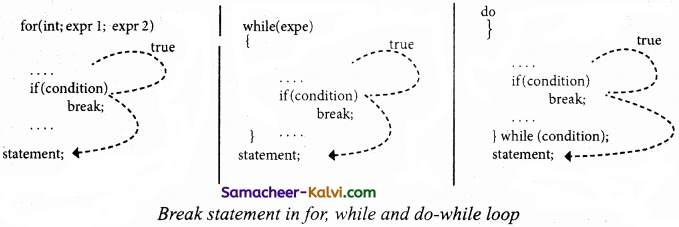Question 26.
What is continue statement? Give the work flow of a continue statement in different statements.
The continue statement works quite similar to the break statement. Instead of terminating the loop (break statement), continue statement forces the loop to continue or execute the next iteration.
The following Figure describes the working flow of the continue statement: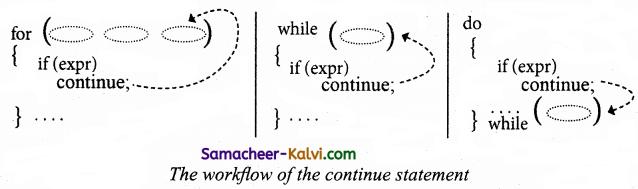Question 27.
Write a C++ program to get the following output: 1 2 3 4 5 7 8 9 10
#include
using namespace std;
int main ( )
for(int i = 1; i<= 10; i++)
{
if (i == 6) continue; else
cout«i« ” “;
}
return 0;
}
Output:
1 2 3 4 5 7 8 9 10Question 28.
Differentiate break and continue statement?

 Break Continue Break is used to terminate the execution of the loop. Continue is not used to terminate the execution of loop. It breaks the iteration. It skips the iteration. When this statement is executed, control will come out from the loop and executes the statement immediately after loop. When this statement is executed, it will not come out of the loop but moves/jumps to the next iteration of loop. Break is used with loops as well as switch case. Continue is only used in loops, it is not used in switch case.

Question 29.
What will be output for the given code below?
#include
using namespace std;
int main( )
{
int count=0; do
{
cout<<“count:”<<count<<endl; count ++; if(count>5)
{
break;
}
}while(count<20);
return 0;
}
Output:
count : 0
count : 1
count : 2Question 30.
Write a C++ program to display number from 10 to 1 using do-while loop.
#include
using namespace std;
int main( )
{
int n = 10;
do
{
cout<<n<<“,”; n–; }while(n > 0);
}
Output;
10, 9, 8, 7, 6, 5, 4, 3, 2, 1

Question 31.
What do you mean by the body of the loop?
The body of the loop:

1. A statement or set of statements forms a body of the loop that are executed repetitively.
2. In an entry-controlled loop, first the test- expression is evaluated and if it is non-zero, the body of the loop is executed otherwise the loop is terminated,
3. In an exit-controlled loop, the body of the loop is executed first then the test- expression is evaluated.Question 32.
Explain if and if-else statement with suitable example.
if statement:
The if statement evaluates a condition, if the condition is true then a true-block (a statement or set of statements) is executed, otherwise the true-block is skipped. The general syntax of the if statement is:
if (expression)
true-block;
statement-x;

In the above syntax, if is a keyword that should contain expression or condition which is enclosed within parentheses. If the expression is true (non-zero) then the true-block is executed and followed by statement-x are also executed, otherwise, the control passes to statement-x. The true-block may consists of a single statement, a compound statement or empty statement. The control flow of if statement and the corresponding flow chart is shown below.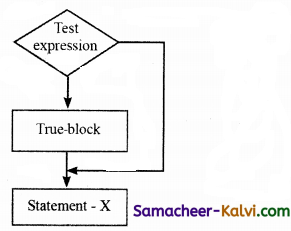C++ Program to check whether a person is eligible to vote using if statement.
#include
using namespace std;
int main( )
{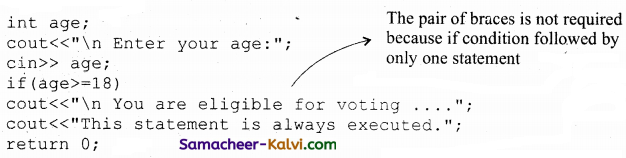}
Output:
You are eligible for voting….
This statement is always executed.

if-else statement:
In the above example of if, allows to execute a set of statement if a condition evaluates to true. What if there is another course of action to be followed if the condition evaluates to false. There is another form of if that allows for this kind of either or condition by providing an else clause. The syntax of the if-else statement is given below:
if(expression)
{
True-block;
}
else
{
False-block;
}
Statement-x
In if-else statement, first the expression or condition is evaluated either True of false.
If the result is true, then the statements inside true-block is executed and false-block is skipped. If the result is false, then the statement inside the false-block is executed i.e., the true-block is skipped.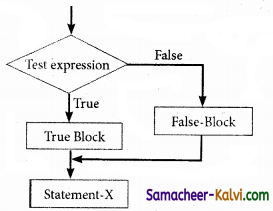C++ program to find whether the given number is even number or odd number using if-else statement.
#include
using namespace std;
int main( )
{
int num, rem;
cout<<“\n Enter a number:”; cin>>num;
rem = num%2;
if(rem==0)
cout<<“\n The given number”<<num<“is Even”;
else
cout<<“\n The given number”<<num<<“is Odd”;
return 0;
}

Output:
Enter number: 10
The given number 10 is Even
In the above program, the remainder of the given number is stored in rem. If the value of rem is zero, the given number is inferred as an even number otherwise, it is inferred as on odd number.Question 33.
Give the key difference between if-eise and switch.
“if-else” and “switch” both are selection statements. The selection statements, transfer the flow of the program to the particular block of statements based upon whether the condition is “true” or “false”. However, there are some differences in their operations. These are given below:
Key Differences Between if-else and switch:

 if else switch Expression inside if statement decide whether to execute the statements inside if block or under else block. expression inside switch statement decide which case to execute. An if else statement uses multiple statements for multiple choices. switch statement uses single expression for multiple choices. If esle statement checks for equality as well as for logical expression. switch checks only for equality. The if statement evaluates integer, character, pointer or floating point type or Boolean type. switch statement evaluates only character or a integer data type. Sequence of execution is like either statement under if block will execute or statements under else block statement will execute. The expression in switch statement decide which case to execute and if do not apply a break statement after each case it will execute till the end of switch statement. If expression inside if turn out to be false, statement inside else block will be executed. If expression inside switch statement turn out to be false then default statements are executed. It is difficult to edit if else statements as it is tedious to trace where the correction is required. It is easy to edit switch statements as they are easy to trace.Question 34.
What do you mean by nested if statement? Explain with suitable example.
An if statement contains another if statement is called nested if. The nested can have one of the following three forms.
(i) If nested inside if part
(ii) If nested inside else part
(iii) If nested inside both if part and else part The syntax of the nested if:
If nested inside if part
if (expression-1)
{
if (expression)
{
True_Part_Statements;
}
else
{
False_Part_Statements;
}
}
else .
body of else part;
If nested inside else part
if(expression-1)
{
body of true part;
}
else
{
if(expression}
{
True_Part_Statements;
}
else
{
False_Part_Statements ;
}
}
If nested inside both if part and else part
if (expression)
{
if (expression)
{
True_Part_Statements;
}
else
{
False_ Part_Statements;
}
}
else
{
if (expression)
{
True_Part_Statements;
}
else
{
False_Part_Statements;
}
}
In the first syntax of the nested if mentioned above the expression-1 is evaluated and the expression result is false then control passes to statement-m. Otherwise, expression-2 is evaluated, if the condition is true, then Nested- True-block is executed, next statement-n is also executed. Otherwise Nested-False-Block, statement-n and statement-m are executed.

The working procedure of the above said if..else structures are given as flowchart below: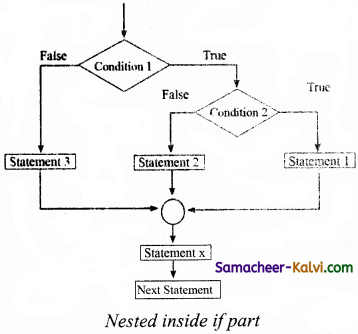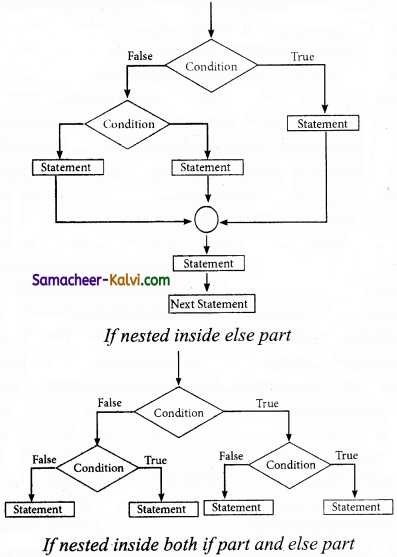C++ program to calculate commission according to grade using nested if statement
#include
using namespace std;
int main ( )
{
int sales, commission;
cout<<”\n Enter Sales amount:”; cin>>sales;
if (sales > 5000)
commission = sales*0 . 10;
cout<<”\n Commission:”<<commission;
}
else
{
commission = sales * 0.05;
cout<<”\n Cornmission:”<< commission;
}
cout<<”\n Good Job . . . . . .“;
return 0;
}
Output:
Enter Sales amount: 6000
Commission: 600
Good Job . . . . . .Question 35.
Write a C++ program to demonstrate switch statement.
#include
using namespace std;
int main ( )
{
{
case ‘A’ : cout<< “\n
Excellent…”;
break;
case ‘B’ :
case ‘C’ : cout<< “\n Welldone . . . .”;
break;
case ‘D’ : cout<< “\n You passed . . . .”;
break;
case ‘ E’ : cout<< “\n Better try again . . . .”;
break;
default : cout<<“\n Invalid Grade . . . .”;
}
return 0;
}
Output:
Welldone . . .Question 36.
Explain in detail the parts of a loop.
Every loop has four elements that are used for different purposes. These elements are:

1. Initialization expression
2. Test expression
3. Update expression
4. The body of the loop

Initialization expression(s):
The control variable(s) must be initialized before the control enters into loop. The initialization of the control variable takes place under the initialization expressions. The initialization expression is executed only once in the beginning of the loop.

Test Expression:
The test expression is an expression or condition whose value decides whether the loop-body will be execute or not. If the expression evaluates to true (i.e., 1), the body of the loop executed, otherwise the loop is terminated. In an entry-controlled loop, the test-expression is evaluated before entering into a loop whereas in an exit-controlled loop, the test-expression is evaluated before exit from the loop.

Update expression:
It is used to change the value of the loop variable. This statement is executed at the end of the loop after the body of the loop is executed.

The body of the loop:
A statement or set of statements forms a body of the loop that are executed repetitively. In an entry-controlled loop, first the test-expression is evaluated and if it is non-zero, the body of the loop is executed otherwise the loop is terminated. In an exit-controlled loop, the body of the loop is executed first then the test-expression is evaluated. If the test-expression is true the body of the loop is repeated otherwise loop is terminated.

Question 37.
Explain the for loop with suitable example.
The for loop is the easiest looping statement which allows code to be executed repeatedly. It contains three different statements (initialization, condition or test-expression and update expression(s)) separated by semicolon.
The general syntax is:
for(initialization (s); test- expression; update expression(s))
{.
Statement 1;
Statement 2;
……….
}
Statement – x;

The initialization part is used to initialize variables or declare variable which are executed only once, then the control passes to test-expression. After evaluation of test- expression, if the result is false, the control transferred to statement-x. If the result is true, the body of the for loop is executed, next the control is transferred to update expression. After evaluation of update expression part, the control is transferred to the test-expression part. Next the steps 3 to 5 is repeated. The workflow of for loop and flow chart are shown below.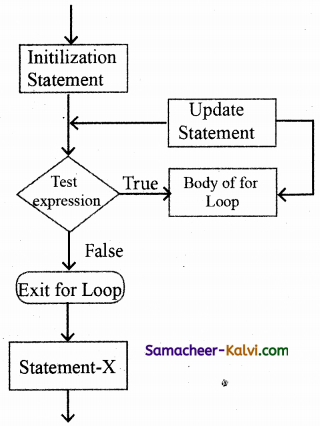C++ program to display numbers from 0 to 9 using for loop.
#include
using namespace std;
int main( )
{
int i;
for(i=0;i<10;i++)
cout<<“value of i :”<<i<<endl;
return 0;
}
Output:
value of i : 0
value of i : 1
value of i : 2
value of i : 3
value of i : 4
value of i : 5
value of i : 6
value of i : 7
value of i : 8
value of i : 9Question 38.
Explain the do-while loop with suitable example.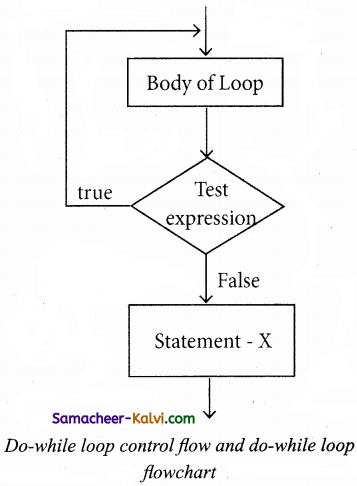The do-while loop is an exit-controlled loop. In do-while loop, the condition is evaluated at the bottom of the loop after executing the body of the loop. This means that the body of the loop is executed at least once, even when the condition evaluates false during the first iteration.
The do-while loop syntax is:
do
{
Body of the loop;
} while(condition);
C++ program to display number from 10 to 1 using do-while loop
#include
using namespace std;
int main( )
{
int n = 10; do
{
cout<<n<<“, “; n–; } while(n>0) ;
}
Output:
10, 9, 8, 7, 6, 5, 4, 3, 2, 1

In the above program, the integer variable n is initialized to 10. Next the value of n is displayed as 10 and n is decremented by 1. Now, the condition is evaluated, since 9 > 0, again 9 is displayed and n is decremented to 8. This continues, until n becomes equal to 0, at which point, the condition n > 0 will evaluate to false and the do-while loop terminates.

Question 39.
Explain the while loop variation.
A while loop may contain several variations. It can be an empty loop or an infinite loop. An empty while loop does not have any statement inside the body of the loop except null statement i.e., just a semicolon.
Eg:
int main ( )
{
int i=0;
while (++i<10000)
→ [This is an empty loop because the while loop does not contain ‘ any statement]
return 0;
}
}
In the above code, the loop is a time delay loop. A time delay loop is useful for pausing the program for some time.
A while loop may be infinite loop when no update statement inside the body of the loop.
Eg:
int main( )
{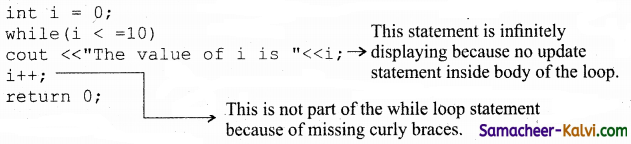}

Similarly, there is another variation of while is also shown below:

int main( )
{
int i=1;
while(++i < 10)
cout<<“The value of i is”<<i;
return 0;
}
In the above statement while (++i < 10), first increment the value of i, then the value of i is compared with 10.
int main( )
{
int i=1;
while(i++ < 10)
cout<<“The value of i is”<<i;
return 0;
}
In the above statement while (i++ < 10), first the value of i is compared with 10 and then the incrementation of i takes place. When the control reaches cout<< “The value of i is”<Question 40.
Write C++ program to solve the following problems.
1. Temperature – conversion program that gives the user the option of converting Fahrenheit to Celsius or Celsius to Fahrenheit and depending upon user’s choice.
#include
using namespace std;
int main( )
{
int res;
double – temp;
cout<,”\n Type 1 to convert fahrenheit to Celsius”
<<“\n Type 2 to convert Celsius to fahrenheit:; cin>>res;
if(res=1)
{
cout<<“Enter temperature in fahrenheit:”; cin>>temp;
cout<<“In Celsius”<<5.0/9.0* (temp-32.0);
}
else
{
cout<<“Enter temperature in Celsius:”; cin>>temp;
cout<<“In fahrenheit”<<9.0/5.0* temp +32.0;
}
cout<<endl;
return 0;
}
Output:
Type 1: to convert fahrenheit to Celsius
Type 2: to covert Celsius to fahrenheit :1 .
Enter temperature in fahrenheit : 120
In Celsius 48.8889

Question 41.
The program requires the user to enter two numbers and an operator. It then carries out the specified arithmetical operation: addition, subtraction, multiplication or division of the two numbers. Finally, it displays the result.
#include
using namespace std;
int main( )
{
char option;
float num1, num2;
cout<<“enter operator either+(or)-(or)* (or)1:”; cin>>option;
cout<<“enter first operand:”; cin>>num1;
cout<<“\n enter second operand:”; cin>>num2;
switch(option)
{
case’+’
cout<<num1+num2;
break;
case’-‘
cout<<num1-num2;
break;
case’*’ ,
cout<<num*num;’
break;
case’/’
cout<<numl/num2;
break;
default:
cout<<“error! Operator is not correct”;
break;
}
return 0;
}
Output:
Enter operator either +(or) – (or) * (or) / : +
Enter first operand: 2
Enter second operand:3
5Question 42.
Program to input a character and to print whether a given character is an alphabet, digit or any other character.
#include
using namespace std;
int main( )
}
char ch;
cout<<“Enter any character”; cin>>ch;
//Alphabet checking condition
if((ch>=’a’ && ch<=’z’) || (ch>=’A’ && ch<= ‘Z’))
{
cout<<ch<<“is an Alphabet”; } else if(ch>=’0′ && ch<=’9′)
{
cout<<ch<<“is a Digit”;
}
else
{
cout<<ch<<“is a special character”;
}
return 0;
}
Output:
Enter any character :8
8 is a Digit

Question 43.
Program to print whether a given character is an uppercase or a lowercase character or a digit or any other character, use ASCII codes for it. The ASCII codes are as given below:

 Characters ASCII Range ‘0’ – ‘9’ 48 – 57 ‘A’ – ‘Z’ 65 – 90 ‘a’ – ‘z’ 97 – 122 Other characters 0 – 255 excluding the above mentioned codes.

#include
using namespace std;
int main( )
{
char ch;
cout<<“Enter any character:”; cin>>ch;
if(ch>=65 && ch<=90)
cout<<endl<<ch<<“uppercase character”; else if(ch>=48 && ch<=57)
cout<<endl<<ch<< “a digit”; else if(ch>=97 && ch<=22)
cout<<endl«ch<<” lowercase character”;
else
cout<<endl<<ch<<“a special character”;
return 0;
}
Output:
Enter any character: D
Uppercase characterQuestion 44.
Program to calculate the factorial of an integer.
#include
using namespace std;
int main( )
{
unsigned int n;
unsigned long factorial =1;
cout<<“enter a integer:”; cin>>n;
for (int i-;i<=n;++i)
{
factorial *=i;
cout<<“factorial of “<<n<<“=”<<factorial;
return 0;
}
Output:
Enter a integer:2
Factorial of 12=479001600

Question 45.
Program that print 1 2 4 8 16 32 64 128.
#include
using namespace std;
int main ( )
{
int j;
for(i=i; i<128; i*=2)
cout<<i<<“ “;
return 0;
)Question 46.
Program to generate divisors of an integer.
#include
using namespace std;
int main( )
(
long int n, j;
cout<<”Enter the number:”; cin>>n;
cout<<endl<<”Divisor of”<> “are”;
for(i = i; i<=n;++i)
{
if (n%i==0)
cout<<” d “<<j;
)
return 0;
Output:
Enter the number : 6
Divisors of 6 are 1 2 3 6

Question 47.
Program to print fibonacci series i.e., 0 1 1 2 3 5 8 ….
#include
using namespace std;
int main( )
mt n1=0, n2=1, n3, i, number;
count<<”enter the number of elements :“; cin>>number;
for (i=0; i<number; i++)
{
n3=n1+n2;
cout<<n3<<” “;
n1=n2;
n2=n3;
}
return 0;
}
Output:
Enter the number of elements:10
0 1 1 2 3 5 13 21 34Question 48.
Programs to produces the following design using nested loops.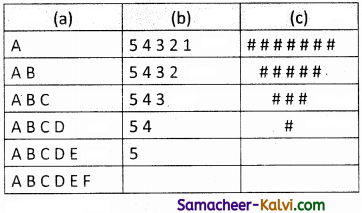(a)
#include
using namespace std;
int main( )
{
char S[ ] = “ABCDEF”
for(int i=0;s[i];++i)
{
for(int j=0;j<=i;++j;
{
cout<<S[j]<<” “;
}
cout<<endl;
}
return 0;
}
Output:
A
A B
A B C
A B C D
A B C D E
A B C D E F

(b)
#include
using namespace std;
int main( )
{
int rows;
for (int i=1; i<=5; ++i) { for (int j =5; j>=i; –j)
{
cout<<j<<” “;
}
cout<<“\n”;
}
return 0;
}
Output:
5 4 3 2 1
5 4 3 2
5 4 3
5 4
5

(c)
#include
using namespace std;
int main( )
{
for (int i=4; i>=1; –i)
{
for (int k=0; k<4-i; ++k)
cout<<” “;
for(int j=i; j<2*i-1; ++j)
{
cout<<“#”;
}
for(j=0;j<i-l;++j) cout«”#”; cout«”\n”;
}
return 0;
}
Output: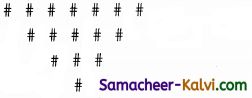Question 49.
Program to check whether square root of a number is prime or not.
#include
#include using namespace std;
int main( )
{
int x, y=1, z, i;
cout<<“Enter a Number ; cin>>x;
z=sqrt(x);
cout<<“\nSquare Root is : “«z;
if(z==1)
{
cout<<“\nThe Number is Not Prime”;
}
else
{
for(i=2; i<z; i++)
{
if(z%i == 0)
{
y = 0;
break;
}
}
if(y == 1)
{
cout<<“\n”<<z<<“is a Prime Number”;
}
else
{
cout<<“\n”<<z<< “is Not a Prime Number”; } } return 0; } Output: Enter a Number: 16 Square Root is: 4 4 is Not a Prime Number.

Question 50.
What is a null statement and compound statement?
The “null or empty statement” is a statement containing only a semicolon. It takes the flowing form: ; // it is a null statement Compound (Block) statement: C++ allows a group of statements enclosed by pair of braces { }. This group of statements is called as a compound statement or a block. The general format of compound statement is: { statement1; statement2; statement3; } For example { int x, y; x = 10; y = x + 10; }Question 51.
What is selection statement? Write it’s types?
The selection statement means the statement (s) are executed depending upon a condition. If a condition is true, a true block (a set of statements) is executed otherwise a false block is executed. The types of selection statement are:

1. if statement
2. if-else statement
3. Nested if statement
4. Switch statement

Question 52.
Correct the following code segment:
if(x=1) p=100;
else P=10;
if (x==1) p=100;
else p-10;

Question 53.
What will be the output of the following code: int year; cin>>year;
if (year % 100==0) ,
if (year% 400==0)
cout<<“Leap”;
else
cout<<“Not Leap year”;

If the input given is (i) 2000 (ii) 2003 (iii) 2010?
(i) I/p: 2000
O/p: Leap

(ii) I/p: 2003
O/p: No output

(iii) I/p: 2010
O/p: No outputQuestion 54.
What is the output of the following code?
for(int i=2; i<=10; i+=2)
cout<<i;
output:
246810

Question 55.
Write a for loop that displays the number from 21 to 30.
for(int i=21; i<=30; i++)
cout<<i;

Question 56.
Write a while loop that displays numbers 2, 4 ,6, 8 ……20.
int i=2;
while(i<=20)
{
cout<<i<<“, i+=2 }

Question 57.
Compare an if and a? : operator
The conditional operator is an expression rather than a statement. There are few places the conditional operator can be used where an if/else cannot be used.Question 58.
Convert the following if-else to a single conditional statement:
if (x>=10)
a=m +5;
else
a=m;
a=(x>=10) ?m+5:m;

Question 59.
Rewrite the following code so that it is functional:
v=5 .
do;
{
Total+=v;
Cout<<total;
while v<=10
int v=5, total=0;
do
{
total += v;
cout<<total;
v=v+1;
while(v<=10);

Question 60.
Write a C++ program to print multiplication table of a given number.
#include
using namespace std;
int main( )
{
int num;
cout<<“Enter number to find multiplication table”; cin>>num;
for(int a=1; a<=10; a++)
{
cout<<num<< ” + ” <<a<< ” = ” <<num*a<<endl;
}
return 0;
}
Output:
Enter number to find multiplication table 3
3 * 1 = 3
3 * 2 = 6
3 * 3 = 9
3 * 4 = 12
3 * 5 = 15
3 * 6 = 18
3 * 7 = 21
3 * 8 = 24
3 * 9 = 27
3 * 10 = 30
Press any key to continueQuestion 61.
Write the syntax and purpose of switch statement.
Purpose: It provides an easy way to dispatch execution to different parts of code based on the value of the expression.
The syntax of the switch statement is;
switch(expression)
{
case constant 1:
statement(s);
break;
case constant 2:
statement(s)
break;
default
statement(s);
}

Question 62.
Write a short program to print following series:
(a) 1 4 7 10……40
#include
using namespace std;
int main( )
{
for(i=1;i<=40;i+=3)
cout<< i << ”
}
cout<<endl;
return 0;
}Question 63.
Explain control statement with suitable example.
Control statements are statements that alter the sequence of flow of instructions.
Selection statement: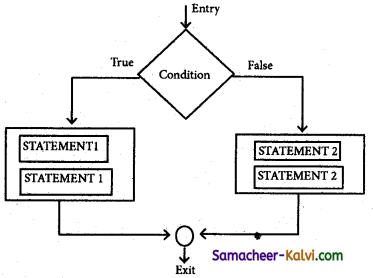In a program, statements may be executed sequentially, selectively or iteratively. Every programming languages provides statements to support sequence, selection (branching) and iteration.

If the Statements are executed sequentially, the flow is called as sequential flow. In some situations, if the statements alter the flow of execution like branching, iteration, jumping and function calls, this flow is called as control flow.
Sequence statement: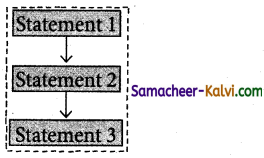The sequential statement are the statements, that are executed one after another only once from top to bottom: These statements do not alter the flow of execution. These statements are called as sequential flow statements. They always end with a semicolon (;).

The selection statement means the statement (s) are executed depending upon a condition. If a condition is true, a true block (a set of statements) is executed otherwise a false block is executed. This statement is also called decision statement or selection statement because it helps in making decision about which set of statements are to be executed.

Iteration statement: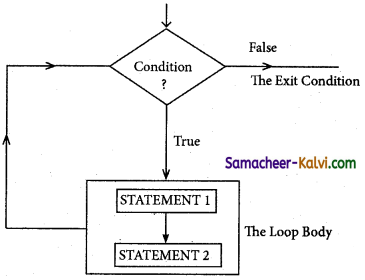The iteration statement is a set of statement that are repetitively executed depends upon a conditions. If a condition evaluates to true, the set of statements (true block) is executed again and again. As soon as the condition becomes false, the repetition stops. This is also known as looping statement or iteration statement. The set of statements that are executed again and again is called the body of the loop.The condition on which the execution or exit from the loop is called exit-condition or test- condition.Question 64.
What is entry control loop? Explain any one of the entry loop with suitable example.
The loop which has a condition check at the entrance of the loop, the loop executes only and only if the condition is satisfied and is called as entry control loop.
Eg: (i) while loop (ii) for loop

while loop:
A while loop is a control flow statement that allows the loop statements to be executed as long as the condition is true. The while loop is an entry-controlled loop because the test-expression is evaluated before the entering into a loop.
The while loop syntax is:
while(Test expression)
{
Body of the loop;
}
Statement-x;
The control flow and flow chart of the while loop is shown below.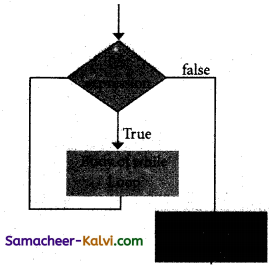In while loop, the test expression is evaluated and if the test expression result is true, then the body of the loop is executed and again the control is transferred to the while loop. When the test expression result is false the control is transferred to statement-x.
#include
using namespace std;
int main( )
{
int i=i,sum=0;
while (i<=10)
{
sum=sum+i;
i++;
}
cout<<“The sum of 1 to 10 is”<<sum;
return 0;
}
Output:
The sum of 1 to 10 is 55

In the above program, the integer variable i is initialized to 1 and the variable sum to 0. The while loop checks the condition, i < 10, if the condition is true, the value of i, which is added to sum and i is incremented by 1. Again, the condition i < 10 is checked. Since 2 < 10, 2 is added to the earlier value of sum. This continues until i becomes 11. At this point in time, 11 < 10 evaluates to false and the while loop terminates. After the loop termination, the value of sum is displayed.Question 65.
Write a program to find the LCM and GDC of two numbers.
#include
using namespace std;
int main( )
{
int a, b, x, y, t, gcd, 1 cm;
cout<<“Enter two Integers\n”; cin>>x>>y;
a=x; ‘
b=y;
while(b!=0)
{
t=b;
b=a%b;
a=t;
}
gcd=a;
1 cm=(x*y)/gcd
cout<<“Greatest common divisor of”<<x<< ” and “<<y<<” = ” <<gcd;
cout<<“Least common multiple of “<<x<<” and “<<y<<” >> ” << 1 cm;
return 0;
}
Output:
Enter two Integers
15
33
Greatest common divisor of 15 and 33=3
Least common multiple of 15 and 33=165

Question 66.
Write program to find the sum of following series:
(i) $$x-\frac{x^{2}}{2 !}+\frac{x^{3}}{3 !}-\frac{x^{4}}{4 !}+\frac{x^{5}}{5 !}-\frac{x^{6}}{6 !}$$

(ii) $$x+\frac{x^{2}}{2}+\frac{x^{3}}{3}+\ldots .+\frac{x^{n}}{n}$$
#include
#include using namespace std;
int main( )
{
int i,n,f=1;
float x, sum=0;
cout<<“\n Enter the value of x and n\n”; cin>>x>>n;
for(i=1;i<=n;i++)
{
f=f*i;
if (i%2==0)
{
sum -= pow (x, i) /f; }
else
{
sum += pow(x,i)/f;
}
}
cout<<“\n sum = “<<sum<<endl;
return 0;
}
Output:
Enter the. value of x and n : 2
6
sum = 0.844444

(ii)
#include .
#include using namespace std;
int main ( )
{
int i,n;
float x, sum=0;
cout<<“\n Enter the value of x and n:\n”; cin>>x>>n;
for (i=i;i<=n;i++)
{
sum+= pow(x,i)/i:
}
cout<<“\n Sum is =” <<sum<<endl;
return 0;
}
Output:
Enter the value of x and n:
2
6
sum is = 27.7333Question 67.
Write a program to find sum of the series S = 1 + x + x2+ +xn
#include
#include using namespace std;
int main( )
{
long i, n, x, sum=1;
cout<<“\n Enter the value of x and n”; cin>>x>>n;
for(i=1;i<=n;i++)
{
sum += pow(x,i)
}
cout<<“\n sum”<<sum;
return 0;
}
Output:
Enter the value of x and n:
5
2
sum 31Question 1.
There are _________ kinds of statements used in C++.
(a) two
(b) three
(c) four
(d) five
(a) two

Question 2.
A group of statements that are enclosed by pair of braces { } are called _______ statement.
(a) compound
(b) single
(c) null
(d) selection
(a) compound

Question 3.
_________ statements are statements that alter the sequence of flow of instructions.
(a) Sequential
(b) Control
(c) Iteration
(d) Selection
(b) ControlQuestion 4.
_________ statement are the statements, that are executed one after another only once from top to bottom.
(a) Control
(b) Compound
(c) Sequential
(d) Empty
(c) Sequential

Question 5.
The sequential flow statements ended with a
(a) .
(b) ;
(c) :
(d) ,
(b) ;

Question 6.
_________ statement are executed depends upon a condition.
(a) Iteration
(b) Selection
(c) Null
(d) Sequential
(b) SelectionQuestion 7.
The ________ statement is a set of statement are repetitively executed depends upon a Condition. .
(a) control
(b) null
(c) selection
(d) iteration
(d) iteration

Question 8.
Iteration statement are also known as _________ statement.
(a) branching
(b) looping
(c) control
(d) empty
(b) looping

Question 9.
The if statement that contains another if statement is called ______ statement.
(a) while
(b) for
(c) do
(d) nested statement.
(d) nested statementQuestion 10.
__________ is a multi – path decision making statement.
(a) if statement
(b) if-else statement
(c) switch statement

Question 11.
The __________ operator is an alternate for ‘if else statement’.
(a) conditional
(b) assignment
(c) logical
(d) arithmetic
(a) conditional

Question 12.
Conditional operator takes __________ arguments.
(a) two
(b) four
(c) five
(d) three
(d) threeQuestion 13.
The conditional operate consists of ________ symbols.
(a) one
(b) two
(c) three
(d) five
(b) two

Question 14.
________ is a conditional operator.
(a) ::
(b) ;
(c) ?
(d) ?:
(d) ?:

Question 15.
________ statement is a multi-way branch statement.
(a) nested if
(b) switch
(d) if-else
(b) switchQuestion 16.
__________ statement reduce the length of code and takes less memory space.
(a) Iteration
(b) Empty
(c) Selection
(d) Sequential
(a) Iteration

Question 17.
C++ supports _______ types of iteration statements.
(a) three
(b) four
(c) two
(d) six
(a) three

Question 18.
Every loop has elements.
(a) five
(b) six
(c) three
(d) four
(d) fourQuestion 19.
In _______ the control variables is initialized.
(a) initialization
(b) test expression
(c) update expression
(d) body of the loop
(a) initialization

Question 20.
________ decides whether the loop-body will be execute or not.
(a) Assignment expression
(b) Test expression
(c) Update expression
(d) Initialization expression
(b) Test expression

Question 21.
__________ expression change the value of the loop variables.
(a) Update
(b) Change
(c) Initialization
(d) Test
(a) UpdateQuestion 22.
The ________ loop is the easiest looping statement which allows code to be executed repeatedly.
(a) while
(b) do-while
(c) for
(d) switch
(c) for

Question 23.
for loop contains __________ different statements.
(a) six
(b) five
(c) four
(d) three
(d) three

Question 24.
Initialization, condition and update expression are separated by:
(a) :
(b) ,
(c) ;
(d) .
(c) ;Question 25.
The multiple initialization and multiple update expressions are separated by:
(a) commas
(b) full stop
(c) semicolon
(d) colon
(a) commas

Question 26.
_______ is an entry check loop.
(a) while
(b) do-while
(c) switch
(d) if
(a) while

Question 27.
The __________ loop is an exit-controlled loop.
(a) for
(b) if
(c) do-while
(d) while
(c) do-whileQuestion 28.
______ are used to interrupt the normal flow of program.
(a) Selection statement
(b) Sequential statement
(c) Control statement
(d) Jump statement
(d) Jump statement

Question 29.
There are _________ types of jump statement.
(a) four
(b) ffye
(c) three
(d) two
(c) three

Question 30.
The ________ statement is a control statement used to transfer the control without any condition.
(a) goto
(b) break
(c) continue
(d) switch
(a) gotoQuestion 31.
for (i = 1; i < 30; i++) how many times the loop will be executed:
(a) 1
(b) 29
(c) 30
(d) 31
(b) 29

Question 32.
if(a>b);
cout<<“greater”;
else
cout<<“lesser”;
What will be the error if thrown by the compiler from above statement:
(a) misplaced if
(b) misplaced else
(c) misplaced if.. .else
(d) misplaced else…if
(b) misplaced else

Question 33. __________ is used to terminate a statement sequence.
(a) case
(b) colon (:)
(c) break
(d) continue
(c) breakQuestion 34.
Identify the incorrect statement in switch case.
(a) Duplicate case values are not allowed
(b) The default statement is not optional
(c) The break statement is optional
(d) Nesting of switch statements is also allowed
(b) The default statement is not optional

Question 35.
________ loop is used in the above program.
(a) while
(b) for
(c) do-while
(d) switch
(c) do-while

Question 36.
An empty while loop does not have any statement inside the body of loop except:
(a) colon
(b) commas
(c) braces
(d) semicolon
(d) semicolonQuestion 37.
In _________ loop the condition is evaluated at the bottom of the loop,
(a) for
(b) while
(c) switch
(d) do-while
(d) do-while

Question 38.
________ statement forces the loop to continue or execute the next iteration.
(a) break
(b) continue
(c) goto
(d) default
(b) continue

Question 39.
________ statement terminates the execution of the loop and resume normal execution after the body of the loop.
(a) if
(b) switch
(c) break
(d) continue
(c) breakQuestion 40.
Identify the invalid one:
(a) if (a > b)
(b) if (a<= b)
(c) if (a= =b)
(d) if a > b
(d) if a > b

Question 41.
Every action in the switch statement should be terminated with a ________ statement.
(a) case
(b) colon
(c) break
(d) continue
(c) break

Question 42.
An _____ loop will be formed if a test – expression is absent in a for loop.
(a) finite
(b) infinite
(c) empty
(d) continue
(b) infiniteQuestion 43.
When a loop has no statement in its body is called an:
(a) while loop
(b) empty loop
(c) finite loop
(d) infinite loop
(b) empty loop

Question 44.
In __________ loop the body of the loop is executed at least once even when the condition is false during first iteration.
(a) do-while
(b) for
(c) while
(d) switch
(a) do-while

Question 45.
Give the output cout<<5+10:
(a) 5+10
(b) 510
(c) 15
(d) 50
(c) 15Question 46.
The loops are enclosed within:
(a) [ ]
(b) { }
(c) <>
(d) ##
(b) { }

Question 47.
From the given code below answer the questions from 47 to 50.
#include
using namespace std,
int main ( )
{
int num = 2
do
cout<<num*num;
num +=1;
}
while(num<6);
}
The control variable used in the program is:
(a) num
(b) +=1
(c) \t
(d) none
(a) numQuestion 48.
The test expression in the program is:
(a) num
(b) num*num
(c) (num<6)
(d) +=1
(c) (num<6)

Question 49.
How many times the loop will be executed?
(a) 2
(b) 4
(c) 5
(d) 3
(b) 4

Question 50.
Match the following:

 (i) Null (a) ?: (ii) if else (b) Goto (iii) While loop (c) Empty (iv) Jump (d) Entry check

(a) (i) – (d); (ii) – (c); (iii) – (b); (iv) – (a)
(b) (i) – (b); (ii) – (a); (iii) – (d); (iv) – (c)
(c) (i) – (a); (ii) – (b); (iii) – (c); (iv) – (d)
(d) (i) – (c); (ii) – (a); (iii) – (d); (iv) – (b)
(d) (i) – (c); (ii) – (a); (iii) – (d); (iv) – (b)Question 51.
Identify the correct statement:
(a) C++ allows a group of statements enclosed by pair Of [ ]
(b) The null statement is a statement containing a set of code.
(c) The sequential statement are the statement that are executed one after another only once from top to bottom.
(d) The iteration statement is a set of statement are not repetitively executed.
(c) The sequential statement are the statement that are executed one after another only once from top to bottom.

Question 52.
Identify the correct statement:
(a) A loop which contains another loop is called as nested loop.
(b) In do-while loop, the condition is evaluated at the top of the loop.
(c) Every loop has four elements that are used for different purpose.
(d) A switch statement can, only work for quality of comparisons.
(b) In do-while loop, the condition is evaluated at the top of the loop.

Question 53.
Choose the odd one out:
(a) for
(b) while
(c) do-while
(d) if-else
(d) if-elseQuestion 54.
Assertion (A):
The iteration statement is a set of statement are repetitively executed depends upon a condition.
Reason (R):
If a condition evaluates to true, the set of statements is executed again and again.
(a) Both A and R are true, and R is the correct explanation for A.
(b) Both A and R are true, but R is not the correct explanation for A.
(c) A is true, but R is false.
(d) A is false, but R is true.
(a) Both A and R are true, and R is the correct explanation for A.

Question 55.
What is the alternate name of null statement?
(a) No statement
(b) Empty statement
(c) Void statement
(d) Zero statement
(b) Empty statement

Question 56.
In C++, the group of statement should enclosed within:
(a) { }
(b) [ ]
(c) ( )
(d) <>
(a) { }Question 57.
The set of statements that are executed again and again in iteration is called as:
(a) condition
(b) loop
(c) statement
(d) body of loop
(d) body of loop

Question 58.
The multi way branching statement:
(a) if
(b) if…else
(c) switch
(d) for
(c) switch

Question 59.
How many types of iteration statements?
(a) 2
(b) 3
(c) 4
(d) 5
(b) 3Question 60.
How many times the following loop will execute? for (int i = 0;i < 10;i++)
(a) 0
(b) 10
(c) 9
(d) 11
(b) 10

Question 61.
Which of the following is the exit control loop?
(a) for
(b) while
(c) do…while
(d) if…else
(c) do…while

Question 62.
Identify the odd one from the keywords of jump statements:
(a) break
(b) switch
(c) goto
(d) continue
(b) switchQuestion 63.
Which of the following is the exit control loop?
(a) do-while
(b) for
(c) while
(d) if-else